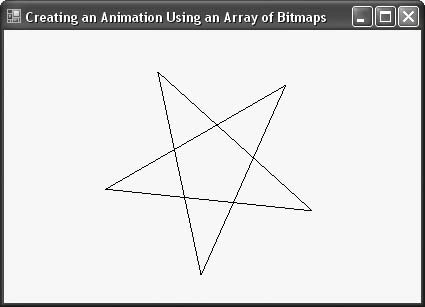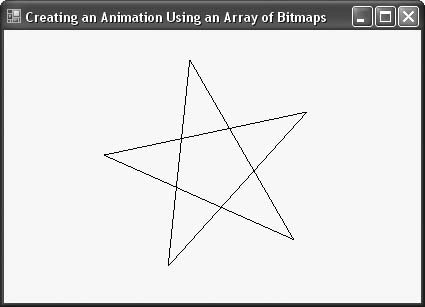# Recipe 10.6. Creating an Animation by Generating Multiple Bitmaps

#### Problem

You want to add a simple animation to your application based on code-drawn bitmaps, but without resorting to complicated video techniques.

#### Solution

Sample code folder: Chapter 10\BitmapAnim

This recipe shows how to create an array of bitmaps in memory, fill them with graphic drawings that vary slightly from one to the next, and then display them in sequence to create an animation.

#### Discussion

This recipe is very similar to the previous one, except that in this case, the images are stored in an array of bitmaps rather than in an ImageList control. The results are very similar.

Create a new Windows Forms application, and add a Timer control named Timer1. Set its Interval property to 50 and its Enabled property to true. Now add the following code to the form's code template:

` Private StarImages(23) As Bitmap Private Sub SpinningStar_Resize(ByVal sender As Object, _       ByVal e As System.EventArgs) Handles Me.Resize    ' ----- Rebuild the images needed for the animation.    Dim xCenter As Integer    Dim yCenter As Integer    Dim radius As Double    Dim canvas As Graphics    Dim counter As Integer    Dim angle As Double    Dim x1 As Single    Dim y1 As Single    Dim x2 As Single    Dim y2 As Single    Const RadPerDeg As Double = Math.PI / 180#    ' ----- Perform some basic calculations.    xCenter = Me.ClientSize.Width \ 2    yCenter = Me.ClientSize.Height \ 2    radius = IIf(Me.ClientSize.Width < Me.ClientSize.Height, _       Me.ClientSize.Width, Me.ClientSize.Height) * 0.4    ' ----- Remove the previous images.    Array.Clear(StarImages, 0, StarImages.Length)    For counter = 0 To StarImages.Length - 1       StarImages(counter) = New Bitmap( _          Me.ClientSize.Width, Me.ClientSize.Height)       canvas = Graphics.FromImage(StarImages(counter))       For angle = 0 To 360 Step 72          x1 = xCenter + radius * _             Math.Cos(RadPerDeg * (angle + counter * 3))          y1 = yCenter + radius * _             Math.Sin(RadPerDeg * (angle + counter * 3))          x2 = xCenter + radius * _             Math.Cos(RadPerDeg * (angle + counter * 3 + 144))          y2 = yCenter + radius * _             Math.Sin(RadPerDeg * (angle + counter * 3 + 144))          canvas.DrawLine(SystemPens.ControlText, _             x1, y1, x2, y2)       Next angle       canvas.Dispose( )    Next counter End Sub `

The code runs every time its form is resized, including once when the form first appears. The 24 bitmap images are recreated nearly instantly, keeping up with the changing form size. Each bitmap is of a five-pointed star, and each star image is rotated slightly from the previous one in the array.

A timer animates the star bitmaps using the 50-millisecond interval set earlier. Add the following code in the timer's Tick event handler to display the next bitmap in the sequence, looping back to the start when the end of the array is reached. The last star is drawn in a position almost rotated to match the first, providing continuously smooth animation:

` Private Sub Timer1_Tick(ByVal sender As System.Object, _       ByVal e As System.EventArgs) Handles Timer1.Tick    ' ----- Draw one of the star array elements.    Dim canvas As Graphics    Static imageNumber As Integer    On Error Resume Next    imageNumber = (imageNumber + 1) Mod StarImages.Length    Try       canvas = Me.CreateGraphics( )       canvas.Clear(Me.BackColor)       canvas.DrawImage(StarImages(imageNumber), 0, 0)       canvas.Dispose( )    End Try End Sub `

The DrawImage() method of the form's Graphics object copies each bitmap onto the form's surface. For maximum smoothness, check that the form's DoubleBuffered property is set to true.

A couple of frames of the rotating star are shown in Figures 10-7 and 10-8. Try resizing the form while the animation is running; you'll see that the star itself resizes as you resize the form.

##### Figure 10-7. Each star bitmap is drawn with a slightly different rotation angle##### Figure 10-8. Multiple bitmaps stored in an array can provide a smooth animation effectVisual Basic 2005 Cookbook: Solutions for VB 2005 Programmers (Cookbooks (OReilly))
ISBN: 0596101775
EAN: 2147483647
Year: 2006
Pages: 400

Similar book on Amazon